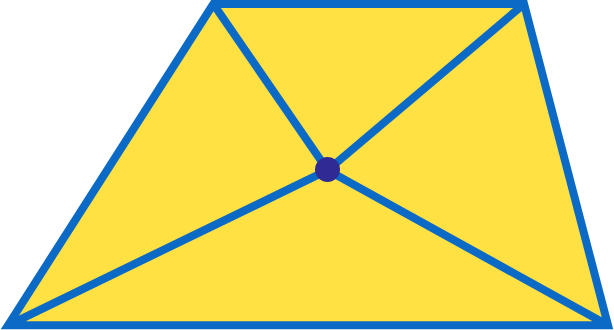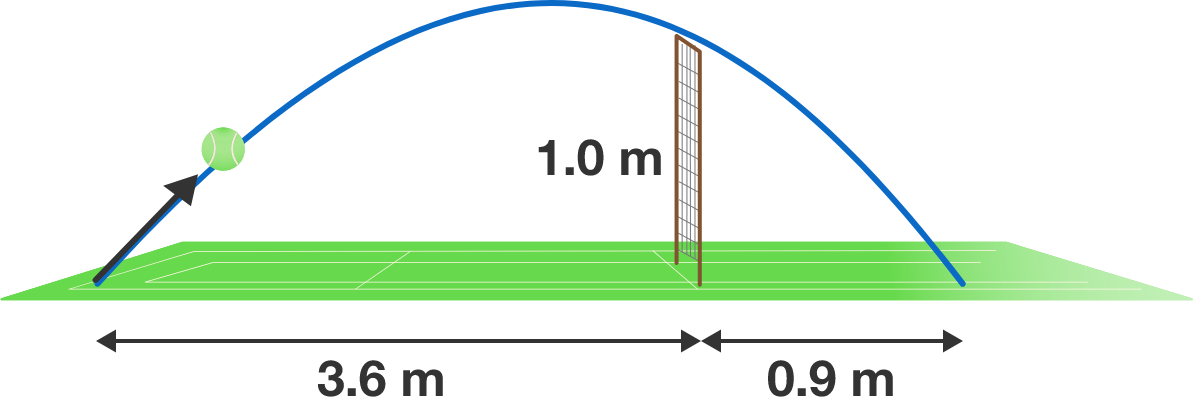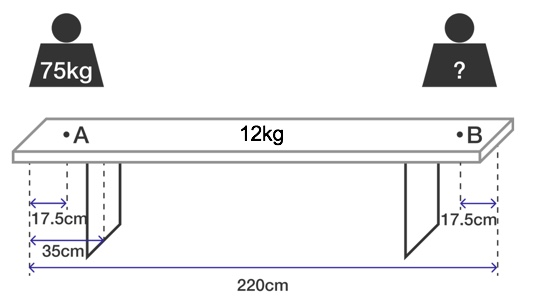# Problems of the Week

Contribute a problem

# 2017-09-18 Intermediate

Given a trapezoid, is it possible to choose a point in the interior that divides the trapezoid into 4 triangles with equal area by drawing segments from the point to the vertices?Note: For this problem, consider a trapezoid to have exactly one pair of parallel sides.

\large \begin{aligned} A = \sqrt{100 }, \quad \quad B = \sqrt{101} \end{aligned} Which is greater, $A$ or $B?$

A "drop shot" in tennis is a shot that barely passes the net and touches the other side of the court close to the net. This makes it difficult for the opponent to reach the ball if the opponent starts running for the ball from the baseline.Suppose you hit a drop shot $3.6\text{ m}$ from your side of the court, making the ball leave your racket very close to the ground. The ball then clears the net at a height of $1\text{ m}$ and touches the ground $0.9\text{ m}$ from the net on the opponent's side, as shown in the diagram.

How long (in seconds) is the ball in the air before hitting the ground after you hit the drop shot, to 2 decimal places?


Details and Assumptions:

• Neglect air resistance.
• The plane containing the trajectory of the ball is perpendicular to the net.
• Use $g = 10 \text{ m/s}^2$.

Define $A$ as follows: $A=1\underbrace{0\cdots 0}_{n \text{ zeroes}}1,$ where $n$ is a positive integer. Does there exist another number $B$ similarly formed that is a multiple of $A$: $B=1\underbrace{0\cdots 0}_{m \text{ zeroes}}1$ such that $B>A?$

Sitting down at the end of an empty ale-bench is usually a bad idea because the bank could overturn immediately. In the figure below, what is the weight needed on point $B$ just enough to compensate a body weight of $75\text{ kg}$ on point $A?$Details and Assumptions:

• The mass of the wooden panel is $m = 12 \,\text{kg}.$
• The length of the bench is $l = 2.2 \, \text{m}$ and its overhang is $35\,\text{cm}$ on each side.
×